## Topic outline

• ### Course Description

####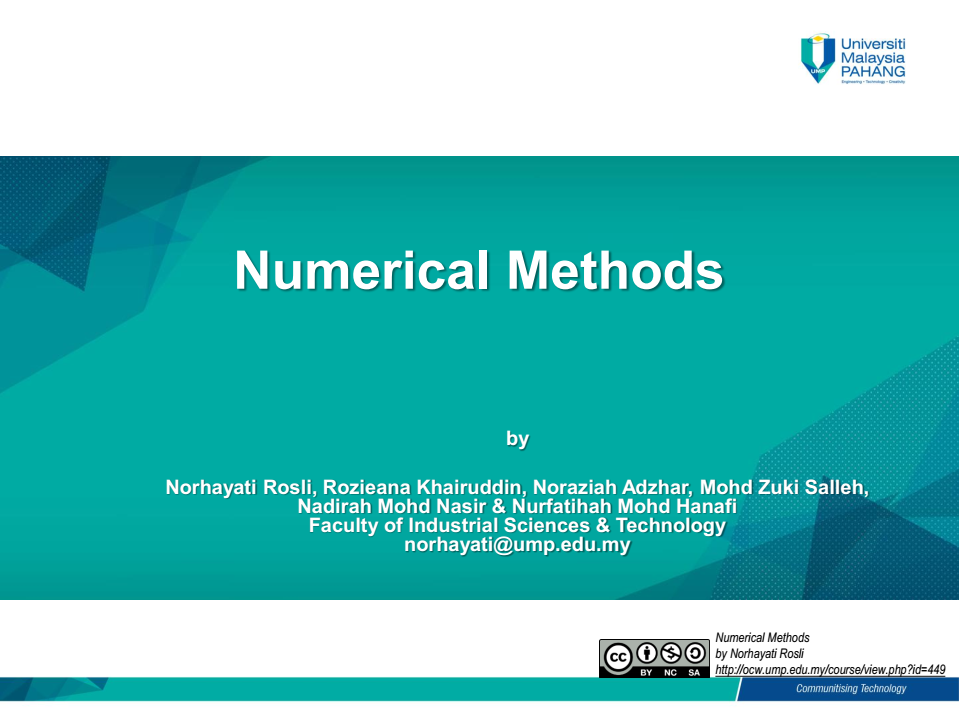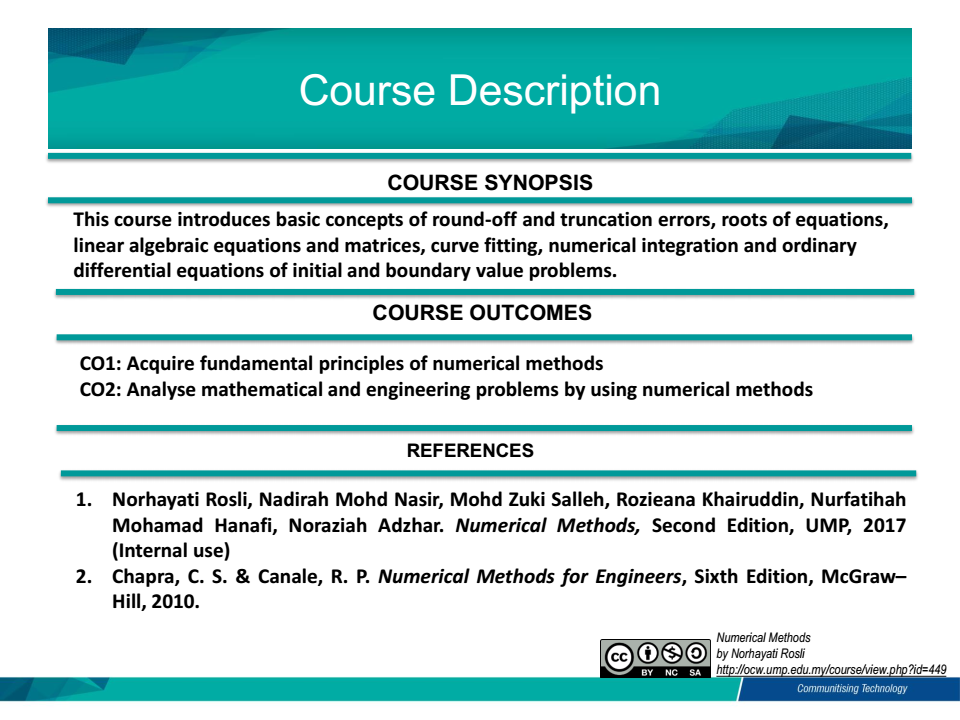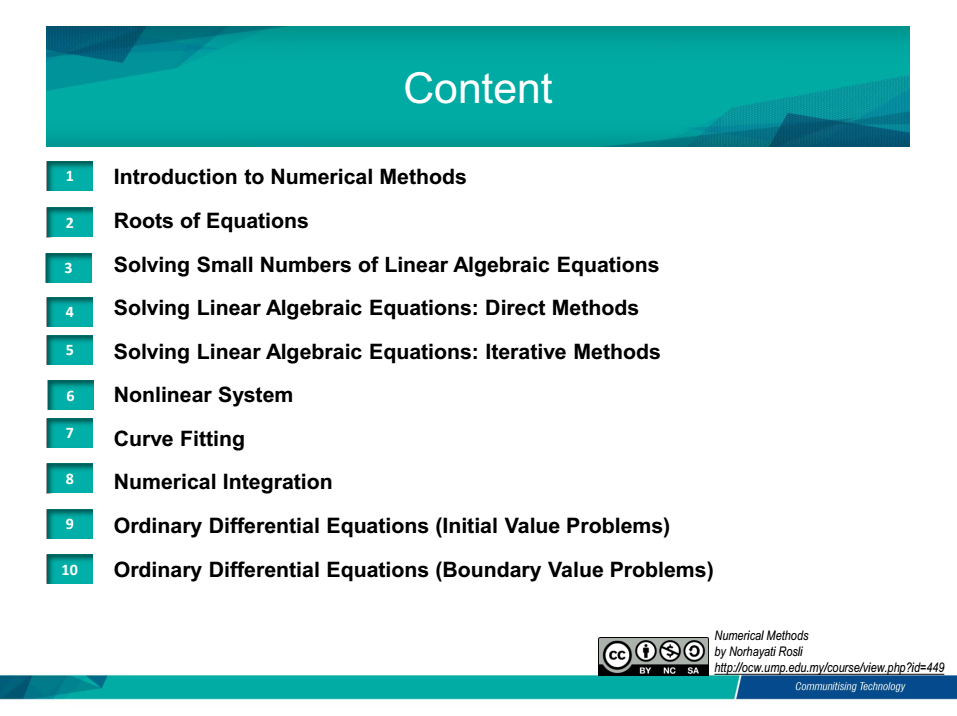•Forum
•• ### Topic 1

#### Introduction to Numerical Methods

1.1 What are Numerical Methods
1.2 Errors
1.3 Taylor's Series Expansion
1.4 Round Off Errors
1.5 Truncation Errors
1.6 Total Numerical Errors

•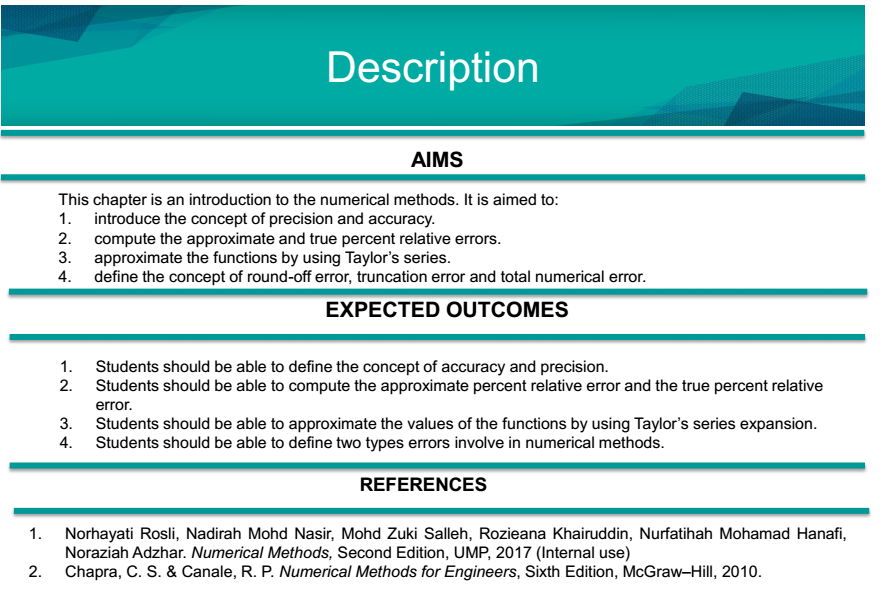•File
• ### Topic 2

#### Roots of Equations

2.1 Introduction
2.2 Graphical Method
2.3 Incremental Search
2.4 Bracketing Methods      2.4.1 Bisection Method      2.4.2 False-Position Method
2.5 Open Methods      2.5.1 Newton Raphson Method      2.5.2 Secant Method
•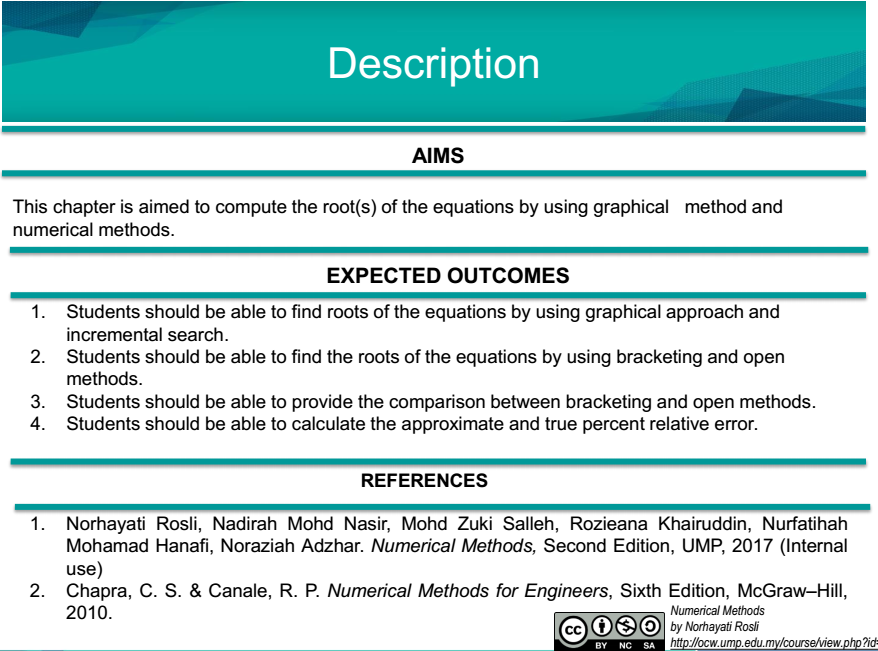•File
• ### Topic 3

#### Solving Small Numbers of Linear Algebraic Equations

3.1 Introduction
3.2 Graphical Method
3.3 Cramer's Rule
•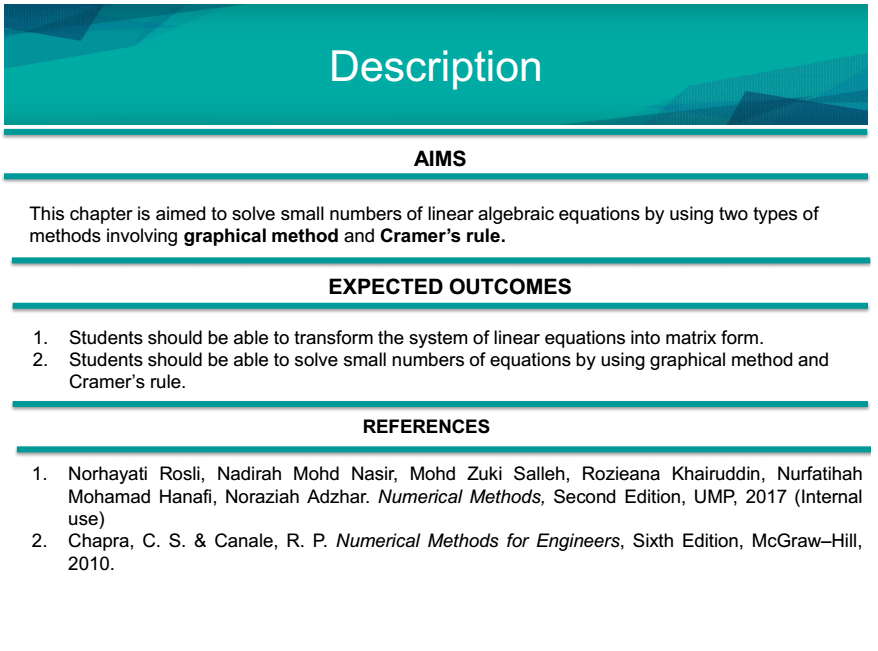•File
• ### Topic 4

#### Solving Linear Algebraic Equations: Direct Methods

4.1 Introduction
4.2 Naive Gauss Elimination Method
4.3 Gauss Elimination Method with Partial Pivoting
4.4 LU Factprization      4.4.1 LU as Gauss Elimination Method      4.4.2 Crout's Method      4.4.3 Cholesky Method
•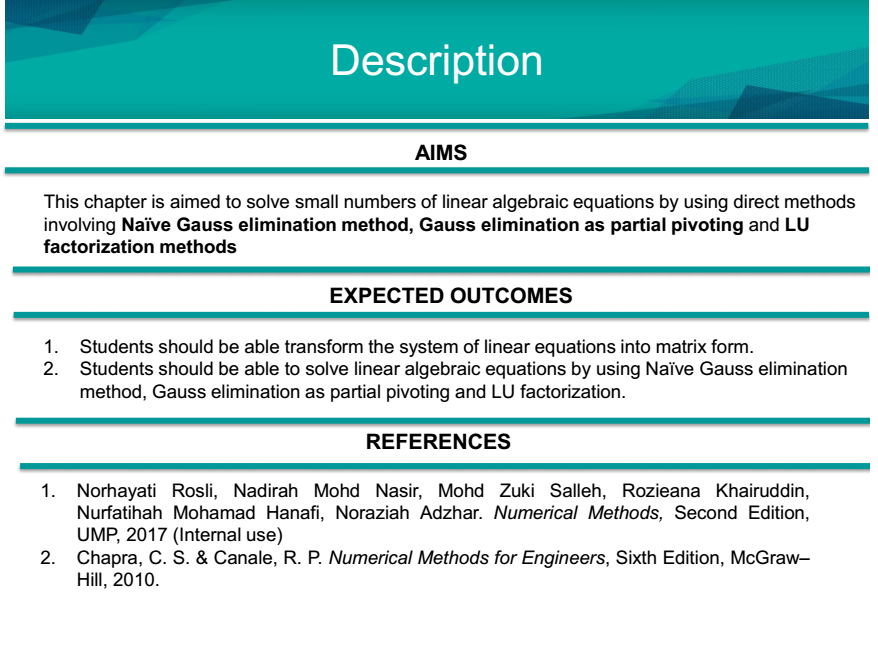•File
• ### Topic 5

#### Solving Linear Algebraic Equations: Iterative Methods

5.1 Introduction
5.2 Jacobi Method
5.3 Gauss Seidel Method
•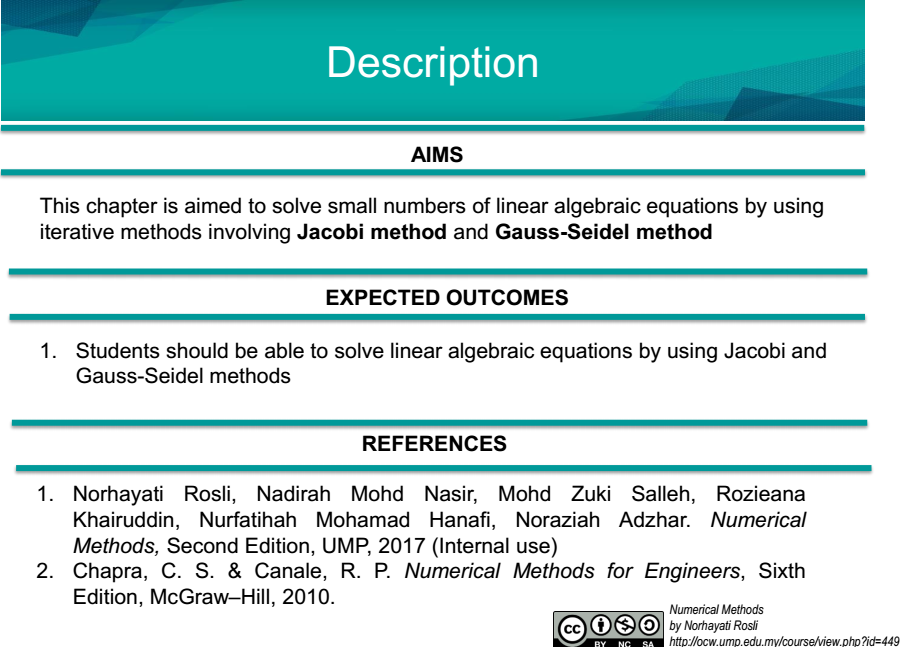•File
• ### Topic 6

#### Nonlinear System

6.1 Introduction
6.2 Newton Raphson Method
•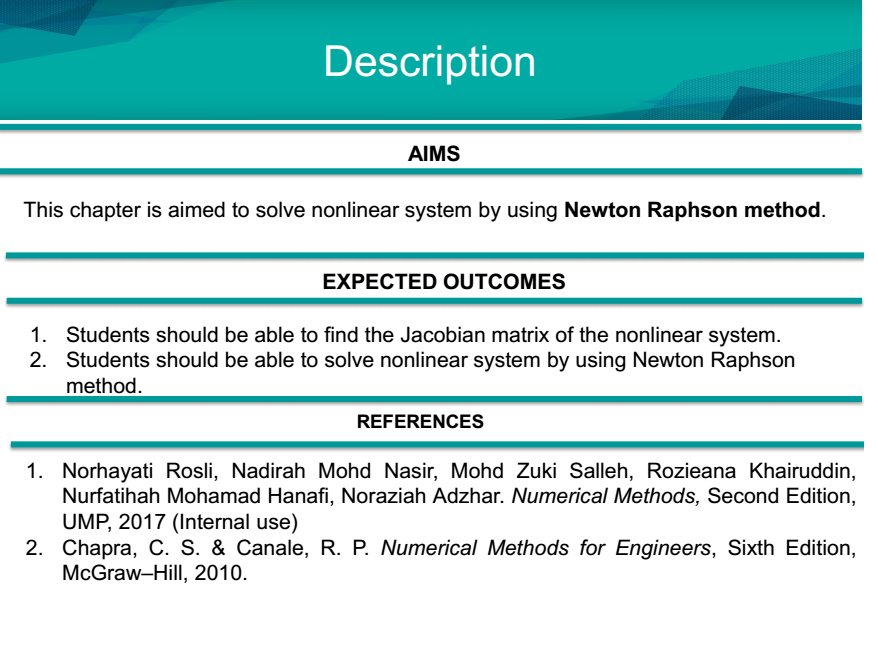•File
• ### Topic 7

#### Curve Fitting

7.1 Introduction
7.2 Polynomial Interpolation      7.2.1 Newton Interpolation Polynomial      7.2.2 Lagrange Interpolation Polynomial      7.2.3 Inverse Interpolation Polynomial
7.3 Splines and Piecewise Interpolation      7.3.1 First Order Spline Interpolation      7.3.2 Second Order Spline Interpolation
•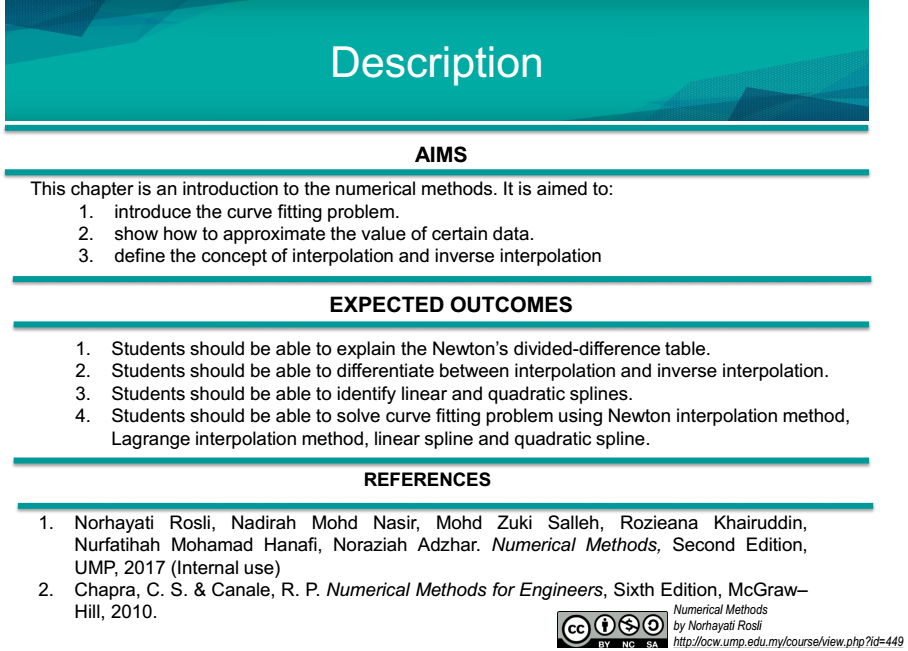•File
• ### Topic 8

#### Numerical Integration

8.1 Introduction
8.2 Numerical Integration Methods       8.2.1 Trapezoidal Rule       8.2.2 Simpson's Rule
•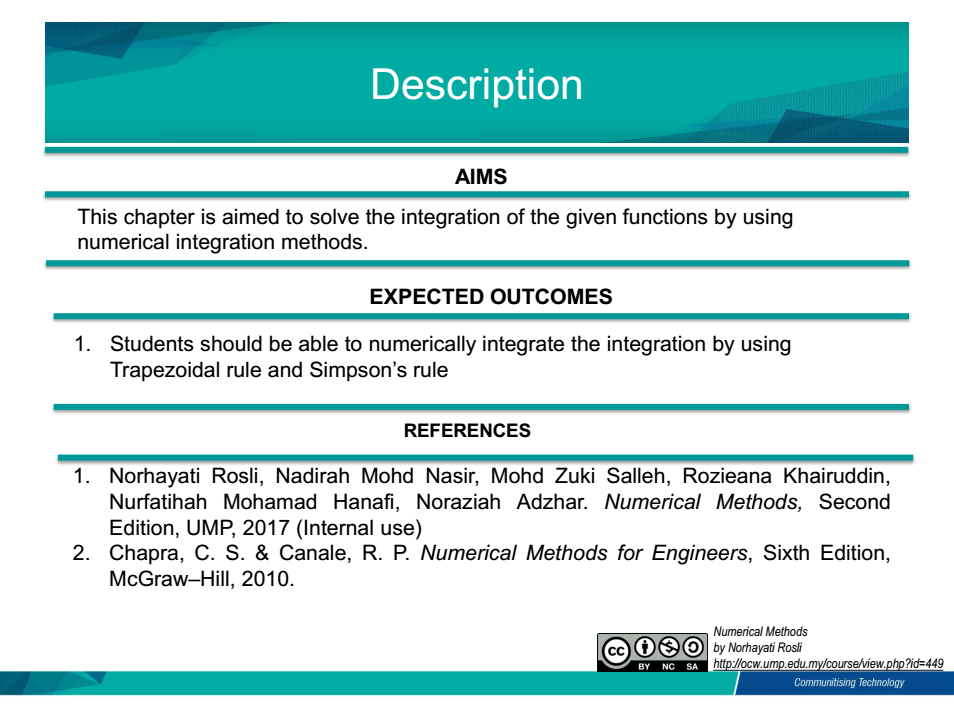•File
• ### Topic 9

#### Ordinary Differential Equations (Initial Value Problems)

9.1 Introduction
9.2 Euler's Method
9.3 Second Order Runge-Kutta Method      9.3.1 Heun Method      9.3.2 Midpoint Method      9.3.3 Ralston Method
9.4 Fourth Order Runge-Kutta Method
9.5 System of Ordinary Differential Equations   9.5.1 Introduction      9.5.2 Euler's Method      9.5.3 Fourth Order Runge-Kutta Method
•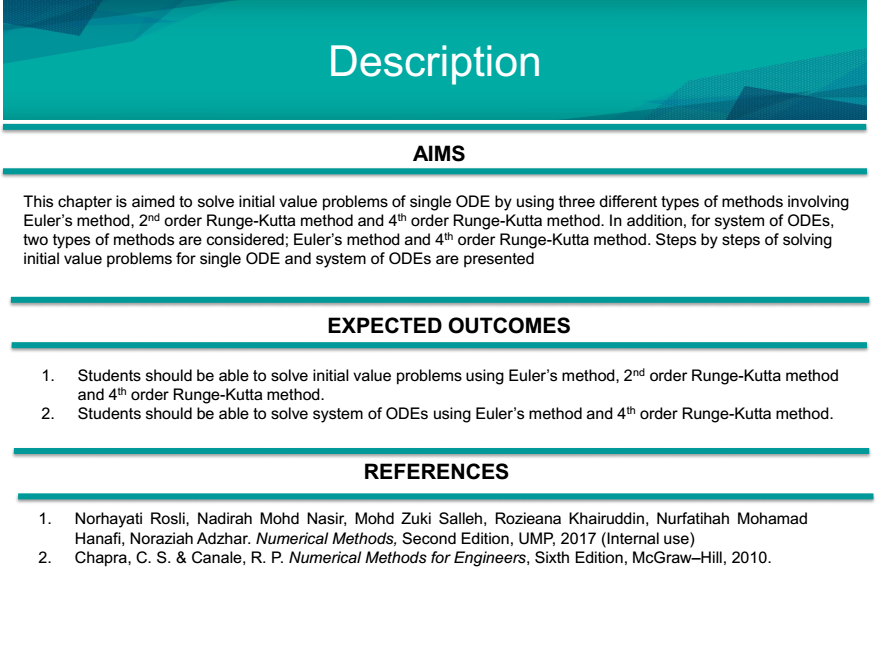•File
• ### Topic 10

#### Ordinary Differential Equations (Boundary Value Problems)

10.1 Introduction
10.2 Shooting Method
10.3 Finite Difference Method
•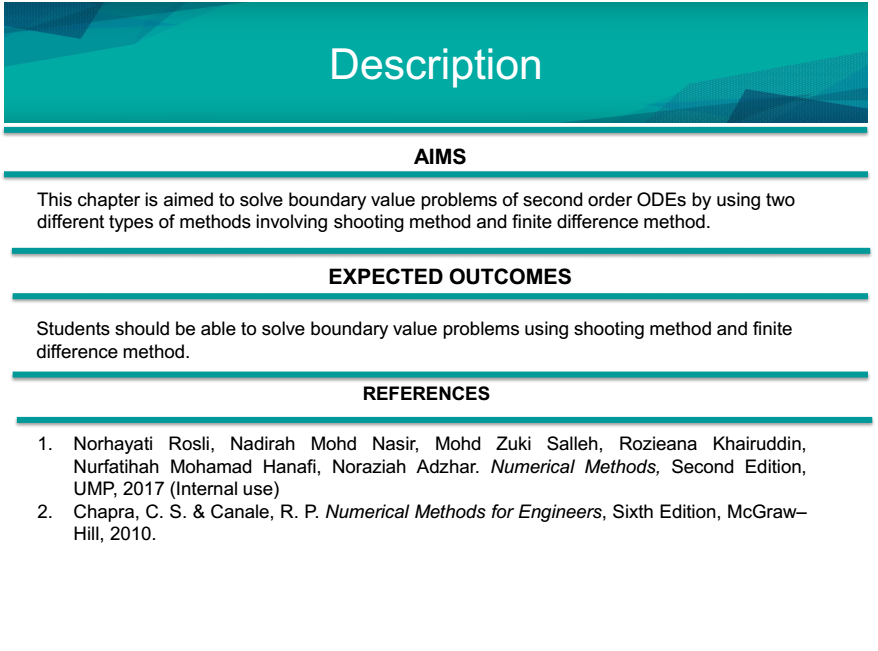•• ### PAST YEAR FINAL EXAM QUESTIONS

PAST YEAR FINAL EXAM QUESTIONS

••••••